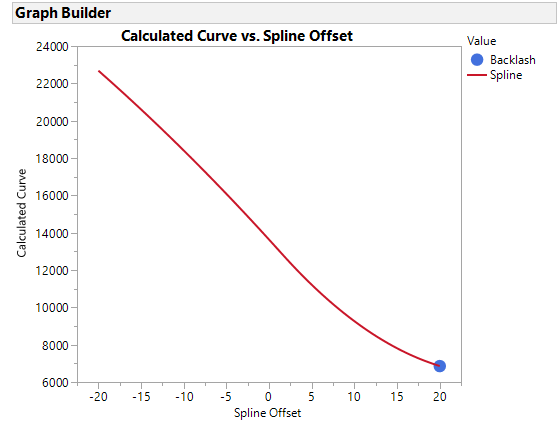Choose Language Hide Translation Bar
Highlighted

## Graph builder with values not in data table

Hello,

I am trying to get my script to graph values that are not in a data table but are generated when the script is executed. The program starts with an import of data in two columns similar to this:

 Position FocusMetric 0 120 -4 140 -8 165 -4 160 -0 130

I then fit a spline up/down the data and determine the offset between the two. The issue comes when I am trying to graph the data. The GraphBuilder doesn't find the variables because they are not in the datatable. Here is my code, everthing seems to work until GraphBuilder. I am trying to get a line graph of offset,splineEval, and a marker for the backlash,splineMin. Any other suggestions are appreciated, this is a new language for me!

``````//Setup arrays for creating spline over data
x = Index(-100,0,1);
offset = Index(-20,20,0.1);

//Determine position minimum index
rPosMin = dt << GetRowsWhere(:Position == ColMin(:Position));

//Create spline coeficients over up/down scan of data
splineUp = SplineCoef(:Position[1::rPosMin-1], :FocusMetric[1::rPosMin-1], 1);
splineDown = SplineCoef(:Position[rPosMin+1::NRow()], :FocusMetric[rPosMin+1::NRow()], 1);

//Create array of least squares
for( i=1, i<=length(offset), i++,
s[i] = Sum(Abs(SplineEval(x,splineDown) - SplineEval((x-offset[i]),splineUp))),
);

//Create spline coefficient over regressed data
si = SplineCoef(offset,s,1);

//Find minimum from regressed data and determine backlash
rSplineMin = Loc(SplineEval(offset,si) == Minimum(SplineEval(offset,si)));
backlash = offset[rSplineMin];
print(backlash);

GraphBuilder(
ShowControlPanel(0),
Variables(
X(offset),
X(backlash, Position(1)),
Y(Values(SplineEval(offset,si))),
Y(rSplineMin, Position(1))
),
Elements(Line(X(1), X(2), Y(1), Y(2), Legend(5)))
);``````
1 ACCEPTED SOLUTION

Accepted Solutions
Highlighted

## Re: Graph builder with values not in data table

JMP Analysis and Grpah platforms require a columns from a reference table.

Create a table from the calculated, offset, backlash, etc. matrix values, then define GraphBuilder using this new table.

4 REPLIES 4
Highlighted

## Re: Graph builder with values not in data table

JMP Analysis and Grpah platforms require a columns from a reference table.

Create a table from the calculated, offset, backlash, etc. matrix values, then define GraphBuilder using this new table.

Highlighted

## Re: Graph builder with values not in data table

Ok I can do that. Once I have them in a datatable is it possible to select one point to make as a marker without having to create a new column for every individual point? I'm trying to put a marker on the minimum point but when I try to set X,Y in GraphBuilder at that index, I get the error "Specified column not found in data table"

``````GraphBuilder(
ShowControlPanel(0),
Variables(
X(:xoff),
X(:xoff[rSplineMin], Position(1)),
Y(:spline),
Y(:spline[rSplineMin], Position(1))

),
Elements(Line(X(1), X(2), Y(1) Y(2), Legend(3))),``````

How would I go about scripting to select that single point and put a marker there?

Highlighted

## Re: Graph builder with values not in data table

I think it would be easier to add the minimum values as a row in the table. The spline points would have a Value of Spline and the extra point a Value of Backlash, then call it out as a special type of  GraphBuilder element.  This curve might look strange because it uses your example data.  The script is attached.Highlighted

## Re: Graph builder with values not in data table

That works. Thanks for your help!

Article Labels

There are no labels assigned to this post.